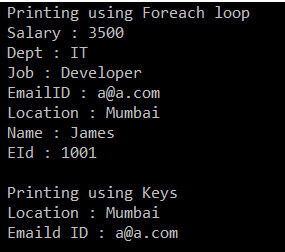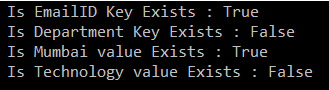# Hashtable in C#

## Hashtable in C# with Examples

In this article, I am going to discuss the Hashtable in C# with examples. Please read our previous article where we discussed the ArrayList Collection in C# with examples. Before understanding the Hashtable in C#, let us first understand the problems that we face with Array and ArrayList collection in C#.

##### Problems with Array and ArrayList Collection in C#:

In the case of Array and ArrayList in C#, we can access the elements from the collection using a key. That key is nothing but the index position of the elements which is starts from zero (0) to the number of elements – 1. But in reality, it’s very difficult to remember the index position of the element in order to access the values.

For example, let’s say we have an employee array that contains the name, address, mobile, dept no, email id, employeeid, salary, location, etc. Now if I want to know the email id or dept no of the employee then it’s very difficult for me. This is because we need to access the value by using the index position. The following example shows this. here we create a collection using ArrayList and then we are accessing the Location and EmailId by using the index position.

```namespace HasntableExample
{
class Program
{
static void Main(string[] args)
{
ArrayList al = new ArrayList();

//I want to print the Location, index position is 4
Console.WriteLine("Location : " + al);

//I want to print the Email ID, index position is 6
Console.WriteLine("Emaild ID : " + al);

}
}
}```

You have a huge number of elements in the collection, then it is very difficult to remember the index position of each element. So, it would not be nice, instead of using the index position of the element, if we can access the elements by using a key. This is where Hashtable in C# comes into the picture.

##### What is a Hashtable in C#?

The Hashtable in C# is a collection that stores the element in the form of “Key-Value pairs”. The data in the Hashtable are organized based on the hash code of the key. The key in the HashTable is defined by us and more importantly, that key can be of any data type. Once we created the Hashtable collection, then we can access the elements by using the keys. The Hashtable class comes under the System.Collections namespace.

Note: The Hashtable computes a hash code for each key. Then it uses that hash code to lookup for the elements very quickly which increases the performance of the application.

Let us see an example for a better understanding of the Hashtable in C#.

```using System;
using System.Collections;

namespace HasntableExample
{
class Program
{
static void Main(string[] args)
{
//Creating Hashtable collection with default constructor
Hashtable ht = new Hashtable();

//Adding elements to the Hash table using key value pair
ht.Add("EId", 1001); //Here key is Eid and value is 1001
ht.Add("Name", "James"); //Here key is Name and value is James

//Printing the keys and their values using foreach loop
Console.WriteLine("Printing using Foreach loop");
foreach (object obj in ht.Keys)
{
Console.WriteLine(obj + " : " + ht[obj]);
}

Console.WriteLine();
Console.WriteLine("Printing using Keys");
//I want to print the Location by using the Location key
Console.WriteLine("Location : " + ht["Location"]);

//I want to print the Email ID by using the EmailID key
Console.WriteLine("Emaild ID : " + ht["EmailID"]);

}
}
}```
###### OUTPUT:When you are working with the Hashtable, then you need to understand two important methods are as follows.

###### ContainsKey() Method:

The ContainsKey() method of the Hashtable is used to check if a given key is present in the Hashtable or not. The following is the syntax for using the ContainsKey() method. If the given key is present in the collection then it will return true else it will return false.

Hashtable.Containskey(key)

###### ContainsValue() Method:

The ContainsValue() Method of the Hashtable class is used to check if a value is present in the Hashtable or not. The following is the syntax for using the ContainsValue() Method. If the given value is present in the collection then it will return true else it will return false.

Hashtable.ContainsValue(value)

Let us see an example for a better understanding of the above two methods:

```using System;
using System.Collections;

namespace HasntableExample
{
class Program
{
static void Main(string[] args)
{
//Creating Hashtable collection with default constructor
Hashtable ht = new Hashtable();

//Adding elements to the Hash table using key value pair
ht.Add("EId", 1001); //Here key is Eid and value is 1001
ht.Add("Name", "James"); //Here key is Name and value is James

//Checking the key using the ContainsKey methid
Console.WriteLine("Is EmailID Key Exists : " + ht.ContainsKey("EmailID"));
Console.WriteLine("Is Department Key Exists : " + ht.ContainsKey("Department"));

//Checking the value using the ContainsValue method
Console.WriteLine("Is Mumbai value Exists : " + ht.ContainsValue("Mumbai"));
Console.WriteLine("Is Technology value Exists : " + ht.ContainsValue("Technology"));